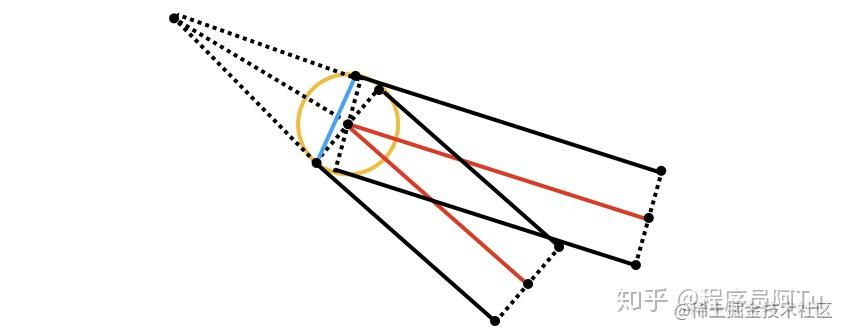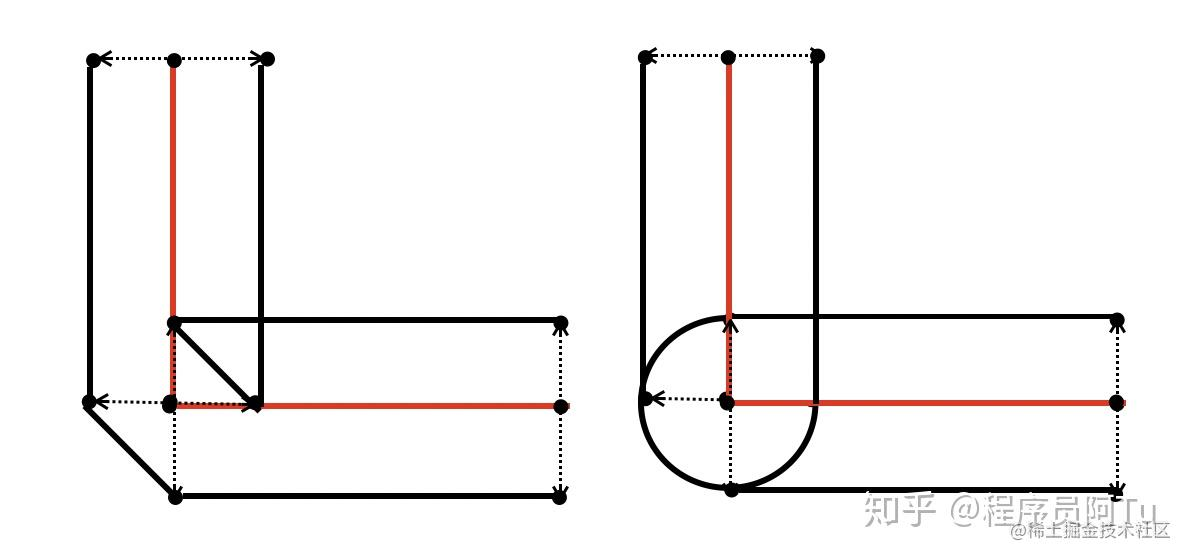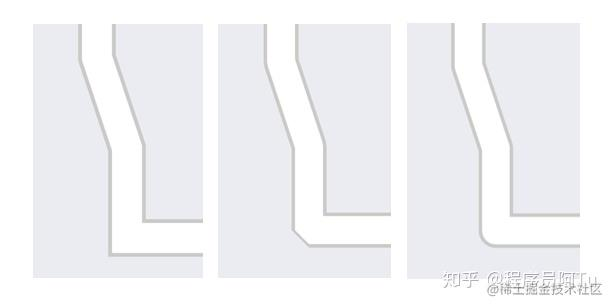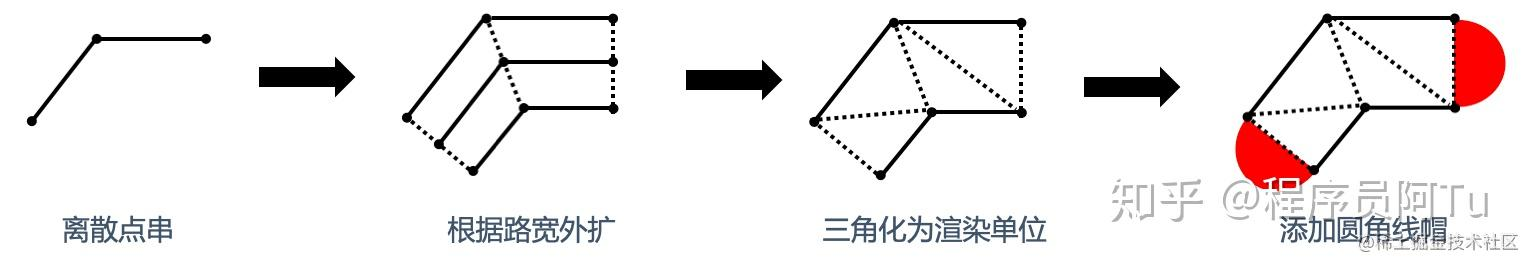# 置顶基于UE4/Unity绘制地图基础元素-线（上篇）精选热门

## 绘制有宽度的线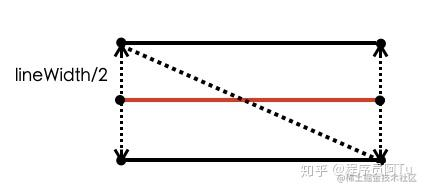• 仅使用相邻点计算垂直向量，导致扩充出的线拐角处会有断裂，如下图所示。可以看到，仅仅每个相邻线段进行扩充是不够的，还需要考虑如何处理线的拐角。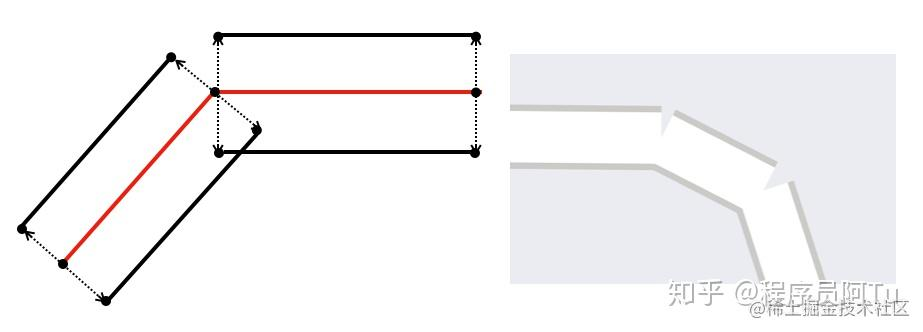• 考虑处理线的拐角，但获取顶点扩充向量的方向和大小不对，导致绘制的线不等宽。下图根据相隔顶点连线的垂线确定扩充向量，但因向量随顶点位置变化而变化，因此不能作为生成等宽线的依据。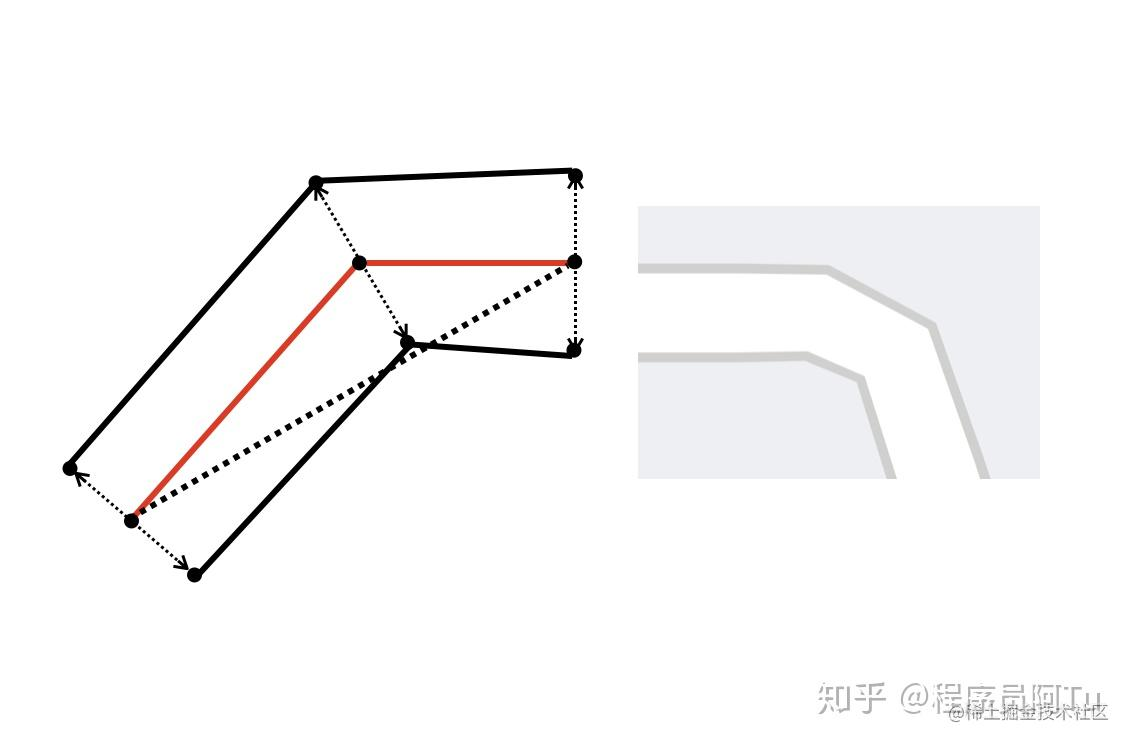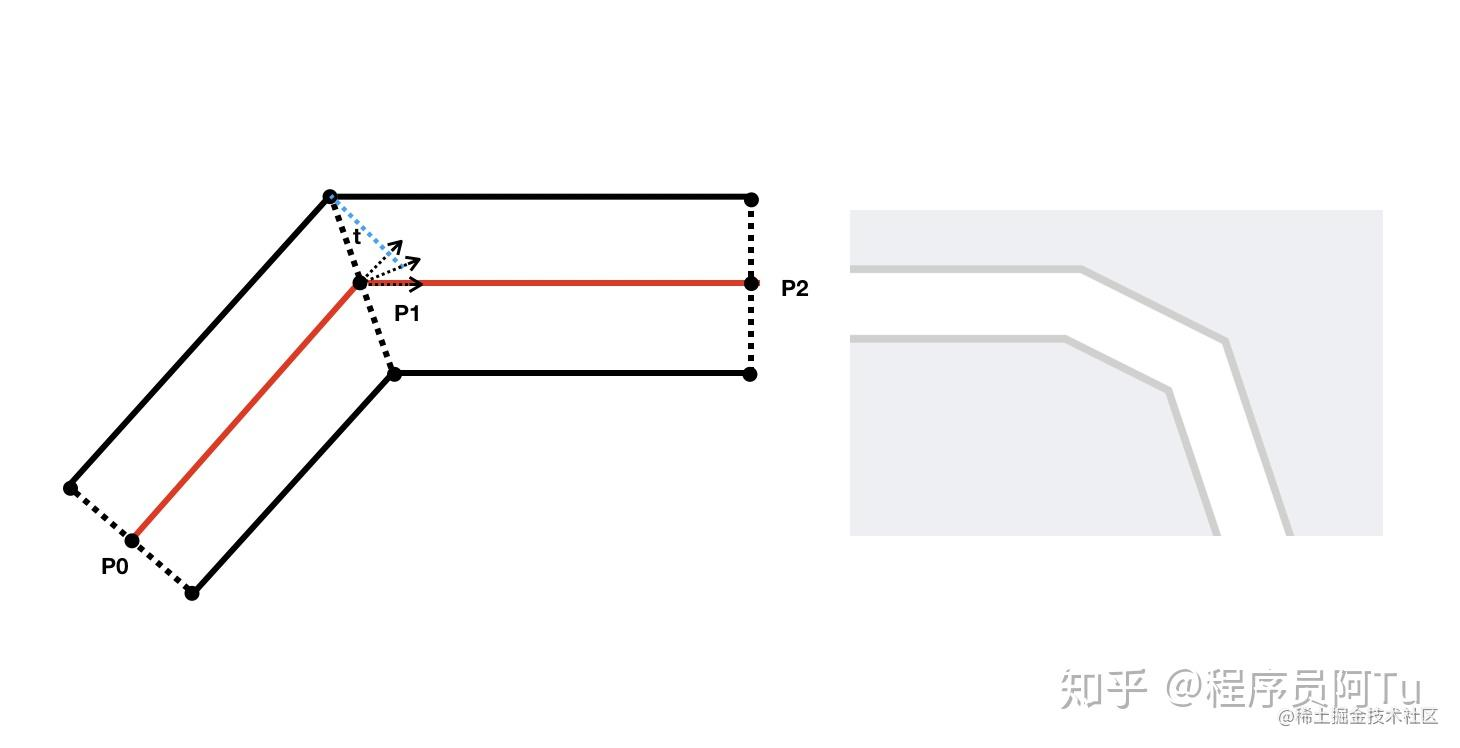``````// 计算扩充方向
Vec2f a = (P1 - P0) * normalized()
Vec2f b = (P2 - P1) * normalized()
Vec2f avg = a + b
Vec2f direction =  Vec2f(-avg.y, avg.x).normalized() //扩充方向为avg的垂直方向

// 计算扩充长度
float t =  Abs(Asin(a × b)) / 2  // 单位向量叉乘获得夹角正弦
float length = lineWidth / 2 / Cos(t)  // 根据角度调整扩充长度
``````

## 绘制线帽LineCap

• Butt 无线帽模式，上一节绘制的线默认即为Butt
• Round 在线的两端添加额外的半圆，其半径为lineWidth/2
• Square 在线两端添加额外的矩形，其高度为lineWidth/2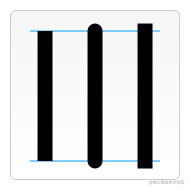Square形式的线帽绘制较为简单，只需要在开头和结尾部分根据延伸方向额外添加矩形即可，两个矩形可以很简单的划分为四个三角形，添加在画线mesh中一同渲染。而Round形式的半圆线帽在绘制上就麻烦了许多，在实践过程中主要探索了以下三个方案：

##### 1、使用三角形近似绘制半圆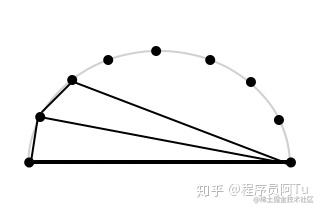##### 2、使用图片近似绘制半圆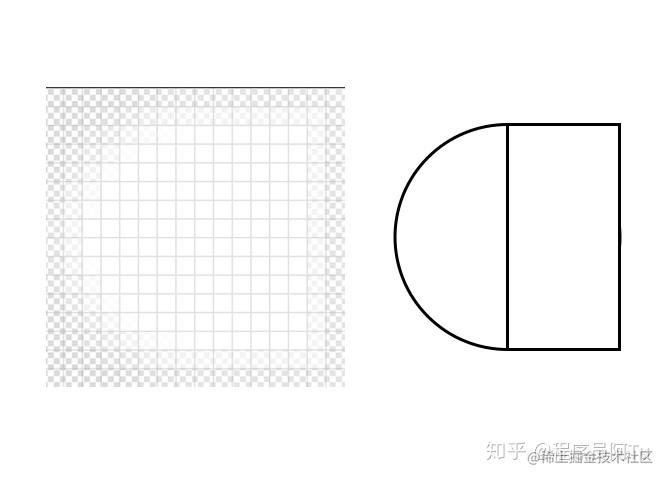##### 3、逐像素绘制半圆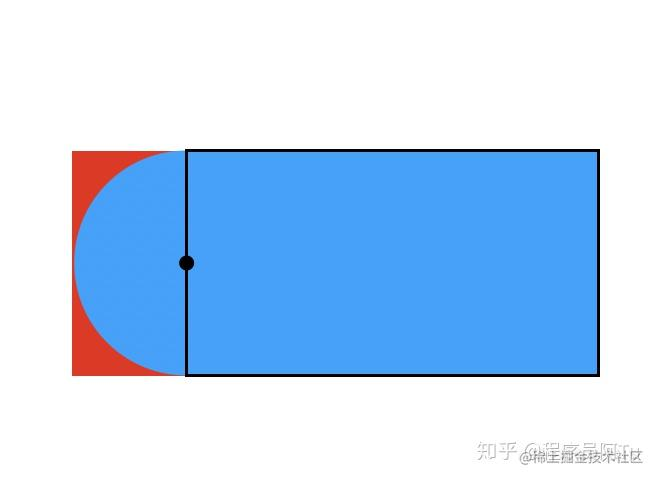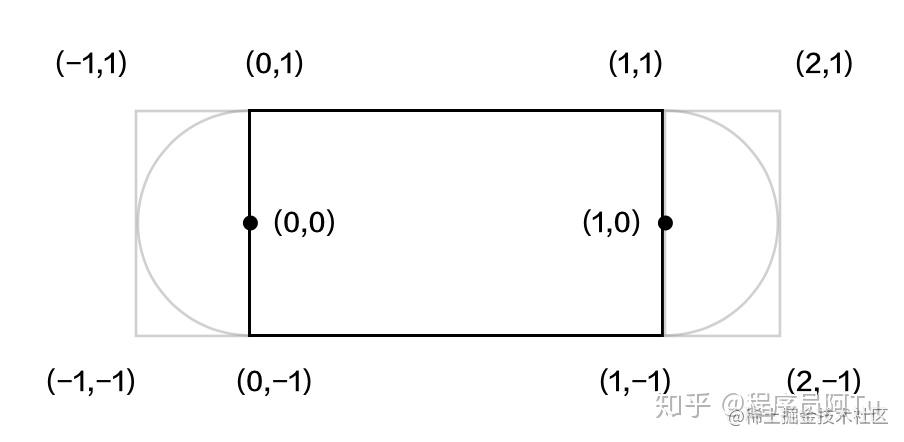``````fixed4 frag (v2f i) : SV_Target
{
if(i.geometryInfo.x < 0)  // 起点侧线帽
{
if(dot(float2(i.geometryInfo.x, i.geometryInfo.y), float2(i.geometryInfo.x, i.geometryInfo.y)) > 1)
{
}
}
else if(i.geometryInfo.x > 1) // 终点侧线帽
{
if(dot(float2(i.geometryInfo.x - 1, i.geometryInfo.y), float2(i.geometryInfo.x - 1, i.geometryInfo.y)) > 1)
{
}
}

return i.color;
}
``````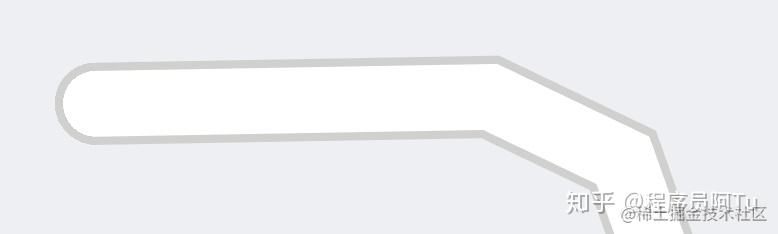## 绘制线拐角LineJoin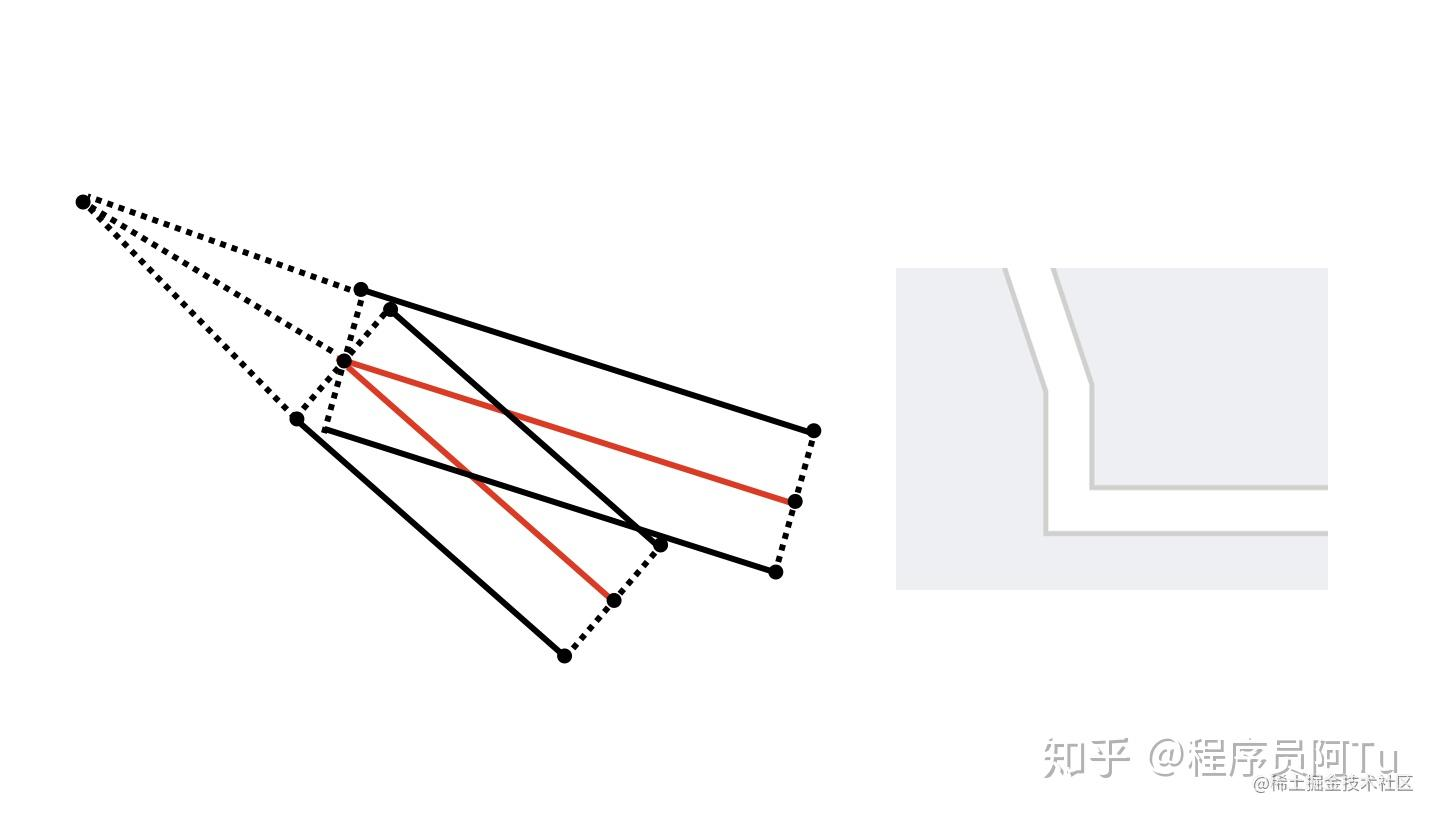• Miter 尖角样式，上一节绘制的线即属于Miter
• Bevel 切角样式，以横切面替代尖角
• Round 圆角样式，以圆弧替代尖角